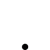# 同一腳本中多個 MACD 跨頻率數值問題？

•   43
• 最後發表   Choc  2 週前
Choc 發文於   2021/06/06

XS 小幫手你好，想請問在同一腳本中 MACD 跨頻率使用時，取得數值的問題~

```input: FastLength(12), SlowLength(26), MACDLength(9);
variable: Freq15("15"), Freq30("30");

variable: difValue15(0), macdValue15(0), oscValue15(0);
variable: difValue30(0), macdValue30(0), oscValue30(0);

xfMin_MACD(Freq15, xfMin_weightedclose(Freq15), FastLength, SlowLength, MACDLength, difValue15, macdValue15, oscValue15);
xfMin_MACD(Freq30, xfMin_weightedclose(Freq30), FastLength, SlowLength, MACDLength, difValue30, macdValue30, oscValue30);

Print("MACD 15分和30分 數值..略...");```

XQ小幫手 發文於   2021/06/08

Hello Choc,

XQ的設定的顯示時間是頻率開始的時間。

30分鐘 Bar 的 09:00 則代表 09:00 到 09:30 之間的資料。

30分鐘 09:00 應該要跟 5分鐘 09:25 比

09:30 應該要跟 09:55 比

Choc 發文於   2021/06/09

```//   分別讀取 15分 和 30分
xfMin_MACD(Freq15, xfMin_weightedclose(Freq15), FastLength, SlowLength, MACDLength, difValue15, macdValue15, oscValue15);
xfMin_MACD(Freq30, xfMin_weightedclose(Freq30), FastLength, SlowLength, MACDLength, difValue30, macdValue30, oscValue30);

// 列印結果
// Date= 2021/06/04 12:45:00 | DIF-15= -2.302545 | MACD-15= -4.199723 |  OSC-15= 1.897178 | DIF-30= -8.694148 | MACD-30= -11.003930 |OSC-30= 2.309781
```

```xfMin_MACD(Freq30, xfMin_weightedclose(Freq30), FastLength, SlowLength, MACDLength, difValue30, macdValue30, oscValue30);

// 列印結果
// Date= 2021/06/04 12:45:00 | -10.899153 | -11.169300 | 0.270147
```

XQ小幫手 發文於   2021/06/09

Hello Choc,

Choc 發文於   2021/06/09

```Date= 2021/06/04 03:45:00 | DIF-15= -4.711676 | MACD-15= -3.943457 | OSC-15= -0.768219 | DIF-30= -8.826208 | MACD-30= -8.536833 | OSC-30= -0.289375
Date= 2021/06/04 04:00:00 | DIF-15= -5.097584 | MACD-15= -4.174282 | OSC-15= -0.923302 | DIF-30= -9.231100 | MACD-30= -8.675686 | OSC-30= -0.555414
Date= 2021/06/04 04:15:00 | DIF-15= -5.980018 | MACD-15= -4.535429 | OSC-15= -1.444589 | DIF-30= -9.869277 | MACD-30= -8.803322 | OSC-30= -1.065955
Date= 2021/06/04 04:30:00 | DIF-15= -6.962209 | MACD-15= -5.020785 | OSC-15= -1.941424 | DIF-30= -10.933000 | MACD-30= -9.229257 | OSC-30= -1.703743
Date= 2021/06/04 04:45:00 | DIF-15= -8.230739 | MACD-15= -5.662776 | OSC-15= -2.567963 | DIF-30= -11.411633 | MACD-30= -9.324984 | OSC-30= -2.086649
Date= 2021/06/04 08:45:00 | DIF-15= -12.620832 | MACD-15= -7.054387 | OSC-15= -5.566445 | DIF-30= -16.079727 | MACD-30= -10.675933 | OSC-30= -5.403795
Date= 2021/06/04 09:00:00 | DIF-15= -13.363830 | MACD-15= -8.316276 | OSC-15= -5.047554 | DIF-30= -17.898551 | MACD-30= -12.120456 | OSC-30= -5.778095
Date= 2021/06/04 09:15:00 | DIF-15= -12.995936 | MACD-15= -9.252208 | OSC-15= -3.743728 | DIF-30= -16.203395 | MACD-30= -11.781425 | OSC-30= -4.421970
Date= 2021/06/04 09:30:00 | DIF-15= -12.080965 | MACD-15= -9.817959 | OSC-15= -2.263006 | DIF-30= -15.636998 | MACD-30= -12.552540 | OSC-30= -3.084459
Date= 2021/06/04 09:45:00 | DIF-15= -10.847516 | MACD-15= -10.023870 | OSC-15= -0.823645 | DIF-30= -15.258081 | MACD-30= -12.476756 | OSC-30= -2.781325
Date= 2021/06/04 10:00:00 | DIF-15= -8.919913 | MACD-15= -9.803079 | OSC-15= 0.883166 | DIF-30= -13.625620 | MACD-30= -12.706529 | OSC-30= -0.9190910
Date= 2021/06/04 10:15:00 | DIF-15= -7.028828 | MACD-15= -9.248229 | OSC-15= 2.219400 | DIF-30= -13.226760 | MACD-30= -12.626757 | OSC-30= -0.6000038
Date= 2021/06/04 10:30:00 | DIF-15= -7.222095 | MACD-15= -8.843002 | OSC-15= 1.620907 | DIF-30= -13.020150 | MACD-30= -12.705435 | OSC-30= -0.3147142
Date= 2021/06/04 10:45:00 | DIF-15= -7.211440 | MACD-15= -8.516690 | OSC-15= 1.305250 | DIF-30= -13.159751 | MACD-30= -12.733356 | OSC-30= -0.4263953
Date= 2021/06/04 11:00:00 | DIF-15= -8.157947 | MACD-15= -8.444941 | OSC-15= 0.286994 | DIF-30= -13.475801 | MACD-30= -12.881845 | OSC-30= -0.5939567
Date= 2021/06/04 11:15:00 | DIF-15= -7.131330 | MACD-15= -8.182219 | OSC-15= 1.050888 | DIF-30= -12.319105 | MACD-30= -12.650506 | OSC-30= 0.331400
Date= 2021/06/04 11:30:00 | DIF-15= -4.969379 | MACD-15= -7.539651 | OSC-15= 2.570272 | DIF-30= -10.921801 | MACD-30= -12.304765 | OSC-30= 1.382964
Date= 2021/06/04 11:45:00 | DIF-15= -3.099254 | MACD-15= -6.651572 | OSC-15= 3.552318 | DIF-30= -10.124080 | MACD-30= -12.145220 | OSC-30= 2.021141
Date= 2021/06/04 12:00:00 | DIF-15= -2.795318 | MACD-15= -5.880321 | OSC-15= 3.085003 | DIF-30= -9.046791 | MACD-30= -11.525535 | OSC-30= 2.478743
Date= 2021/06/04 12:15:00 | DIF-15= -2.365792 | MACD-15= -5.177415 | OSC-15= 2.811623 | DIF-30= -9.325994 | MACD-30= -11.581375 | OSC-30= 2.255382
Date= 2021/06/04 12:30:00 | DIF-15= -2.660428 | MACD-15= -4.674018 | OSC-15= 2.013590 | DIF-30= -9.252553 | MACD-30= -11.115611 | OSC-30= 1.863058
Date= 2021/06/04 12:45:00 | DIF-15= -2.302545 | MACD-15= -4.199723 | OSC-15= 1.897178 | DIF-30= -8.694148 | MACD-30= -11.003930 | OSC-30= 2.309781
Date= 2021/06/04 13:00:00 | DIF-15= -3.591354 | MACD-15= -4.078049 | OSC-15= 0.486695 | DIF-30= -9.575818 | MACD-30= -10.718307 | OSC-30= 1.142490
Date= 2021/06/04 13:15:00 | DIF-15= -3.403482 | MACD-15= -3.943136 | OSC-15= 0.539653 | DIF-30= -8.538781 | MACD-30= -10.510900 | OSC-30= 1.972119
Date= 2021/06/04 13:30:00 | DIF-15= -3.975338 | MACD-15= -3.949576 | OSC-15= -0.025762 | DIF-30= -9.197239 | MACD-30= -10.248168 | OSC-30= 1.050929
```

```Date= 2021/06/04 03:00:00 | -8.109656 | -7.531448 | -0.578208
Date= 2021/06/04 03:30:00 | -8.140692 | -7.653297 | -0.487395
Date= 2021/06/04 04:00:00 | -9.228932 | -7.968424 | -1.260508
Date= 2021/06/04 04:30:00 | -10.813976 | -8.537534 | -2.276441
Date= 2021/06/04 08:45:00 | -13.867056 | -9.603439 | -4.263617
Date= 2021/06/04 09:15:00 | -13.927251 | -10.468201 | -3.459050
Date= 2021/06/04 09:45:00 | -12.718832 | -10.918327 | -1.800505
Date= 2021/06/04 10:15:00 | -12.763873 | -11.287437 | -1.476437
Date= 2021/06/04 10:45:00 | -13.311824 | -11.692314 | -1.619510
Date= 2021/06/04 11:15:00 | -11.834443 | -11.720740 | -0.113703
Date= 2021/06/04 11:45:00 | -10.502199 | -11.477032 | 0.974833
Date= 2021/06/04 12:15:00 | -10.276057 | -11.236837 | 0.960780
Date= 2021/06/04 12:45:00 | -10.899153 | -11.169300 | 0.270147
Date= 2021/06/04 13:15:00 | -11.103583 | -11.156157 | 0.052574 ```

XQ小幫手 發文於   2021/06/10

Hello Choc,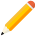發表新主題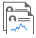我的發文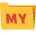我的收藏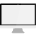分類

Close